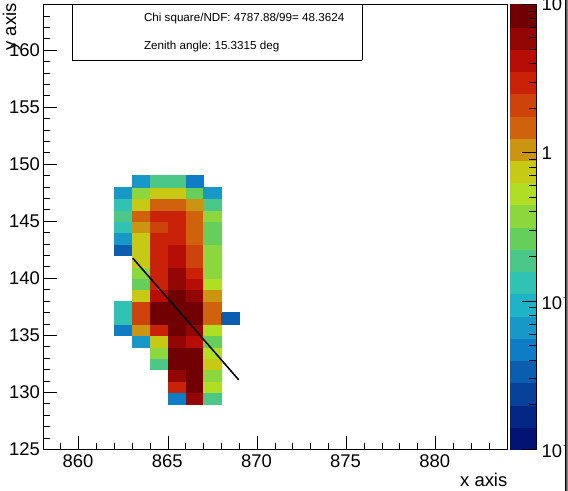# Problem fitting a linear TF1 in a TH2

Hello,

I’m trying to fit multiple TH2 with a straight line, using the command TH2.Fit(“pol1”). While this works, I’m not sure that this is the right way to do it, since it fails when the tracks are completely vertical, as I show in the figure.The histogram is filled from a tree in an external file, I’m uploading a shorter version of this file along with a short version of my code that can be ran and that contains the fitting part.

Code: DrawHistFit.cc (6.2 KB)
Makefile:Makefile.c (709 Bytes)
(NOTE: it wouldn’t let me upload the makefile without an extension, so I added the .c, but the original file has no extension)
External file with tree: TenTracks-ohdu2-run300-Zoomed.root (34.3 KB)

The fitting of the histogram starts at line 152.

I couldn’t find anything yet that migh solve this problem with vertical tracks, I’d appreciate if you could help me with this issue.

Julian.

ROOT Version: 6.14/04
Platform: Ubuntu

Hi,
Unfortunately it will not be possible to fit vertical tracks since you cannot defined as a function `y = f(x)`, but it is instead a function `x=constant`. The easier solution is then rotate the histogram.
What we could do is to add an option to `TH2::Fit` to fit the data as a function `x = f(y)` instead of `y=f(x)`

Lorenzo

So it isn’t possible (yet) to fit with something like an implicit function (ax+by+c=0)? That’s too bad, because I also need to plot some other histograms like that one, but some of them are horizontal.

This would indeed help a lot in doing this

How could I do that, in a way that I can later fit the ‘inverted’ histogram (x->y’, y->x’) with y’=f(x’)?

Thanks for the help!

Hi,

if you are fitting an implicit function as `f(x,y)=0` to some data points (x,y), it is better you do something like in this tutorial, where you create yourself a least-square function to minimise,
see https://root.cern.ch/doc/master/fitCircle_8C.html

The fit of a TH2 histogram to a functions makes a least square function using the difference between the y bin centre and the function value `y = f(x)` , weighted by the y bin width divided by the bin error. This might also be not really appropriate in some cases.

I have created a JIRA item for adding anyway the option to fix a `x = f(y)` function, see
https://sft.its.cern.ch/jira/browse/ROOT-11024

Attached you can find some code example to rotate a 2-dimensional histogram (i.e. to swap the x-y axes)

Best regards

Lorenzo
RotateHistogram.C (810 Bytes)

I’ll check both out, thanks for the help!

Hello!
How did you manage to solve this? I have a similar problem with a fit for a graph.

Raluca